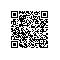# Perspective Devision

OpenGL的Projection矩阵为：

[

n/r, 0, 0, 0,

0, n/t, 0, 0,

0, 0, -(f+n)/(f-n), -2fn/(f-n),

0, 0, -1, 0

]

z' = -(f+n)/(f-n) * z - 2fn/(f-n)

w' = -z

z'' = (f+n)/(f-n) + 2fn/z*(f-n)

w'' = 1

_ZBufferParams.x = (1.0 - far/ near) / 2.0;
_ZBufferParams.y = (1.0 + far/ near) / 2.0;

Linear01Depth = z/f, 即为[0,1]范围内的线性深度。上述算法和Unity中的Linear01Depth函数是一致的。使用钉钉扫一扫加入圈子
+ 订阅